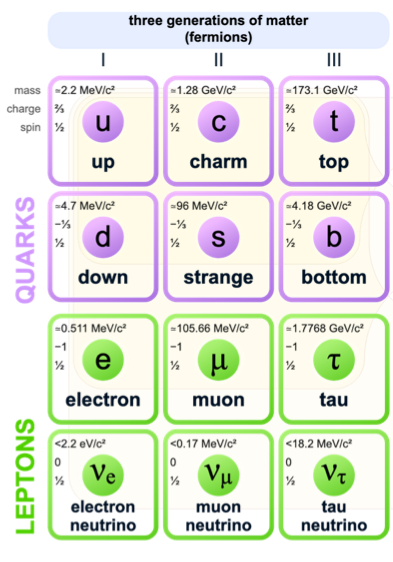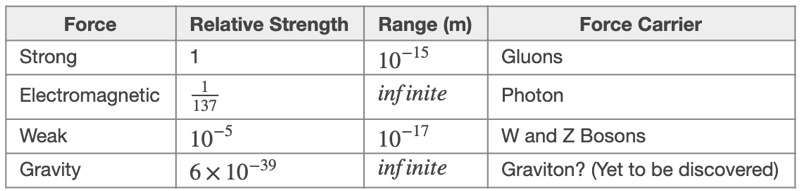# M8-S12a: The Standard Model of Matter

• ### investigate the Standard Model of matter, including:

• quarks, and the quark composition hadrons
• leptons
• fundamental forces (ACSPH141, ACSPH142)

• The discovery of new particles within the nucleus led scientists to propose the Standard Model of Matter.
• Most of the particles in the standard model only exist in high energy situations, such as those created in particle accelerators.
• The Standard Model states that all matter is composed of small elementary particles that exist on their own or in groups to:
1. Form other subatomic particles
and
1. Transmit force (quantum theory states that force acting between objects is caused by force-particles travelling between them.
• There are three types of elementary particles:
• Quarks (matter particles)
• Leptons (matter particles)
• Bosons (force-mediating particles)*Gravitons are force-mediating particles for gravitational force which are yet to be discovered and are typically not included in the Standard Model of Matter.

• Quarks combine together to make other particles, while Leptons do not – both are classified as fermions due to their half integer spin values. Bosons are not classified as fermions because they have whole integer spin values).

• Particles that consist of quark combinations are called hadrons – there are two kinds of hadrons: baryons and mesons. All hadrons are affected by strong force (gluons) because they contain quarks.
• Baryons are hadrons which consist of three quark particles. For example, protons and neutrons are baryons.
• Meson are hadrons which consist of two quark particles (one quark and one anti-quark). For example, pions.

### Generations of Fundamental Particles

• Each fundamental matter particle can be divided into three generations, each based on their abundance and stability. Quarks and leptons in the second and third generations are usually referred to as exotic due to their rarity.• There are two flavours of quarks in each generation. Each flavour has a special name and is distinguished by its charge. For example, in the first generation an up quark has a charge of +2/3 while a down quark has a charge of -1/3.

• Generation I: up and down quarks
• Generation II: charm and strange quarks
• Generation III: top and bottom quarks

Thus, a proton with +1 charge is made of two up and one down quark (uud) while a neutron is made of two down and one up quarks (udd).

• Leptons also have generations but unlike quarks, they do not have flavours.
• Generation I: electron and electron neutrino.
• Generation II: muon and muon neutrino.
• Generation III: tau and tau neutrino.

### Bosons

These fundamental particles are held together by four fundamental forces, which are mediated by bosons:

• Strong nuclear force which holds protons and neutrons together in the nucleus (short range force but strongest amongst others) is mediated by Gluons (g).
• Weak nuclear force which interacts with particles during transmutation through beta decay (short range force) is mediated by W+, W- and Zo gauge bosons. Z bosons are larger than the W±
• Electromagnetic force (long range) which exists between particles is mediated by photons (γ).• Higgs bosons are a group of force-mediating fundamental particles that are scalar in nature. This means they have no direction, no charge, no spin but have mass. In 2013, experimental results from particle colliders confirmed the existence of Higgs bosons which are critical in explaining the detection of mass in W/Z bosons (weak force).

 Advantages/benefits Disadvantages/limitations ·      The model explains the composition of sub-atomic particles and the interaction between them. ·      The model explains how the fundamental forces are mediated – through the understanding of bosons. ·      The model provides a common understanding of force and matter. It is holistic and non-contradictory. ·      The model has predicted the existence and nature of sub-atomic particles such as the muon and the Higgs boson. ·      The model is consistent with several fields of quantum physics including quantum electrodynamics and electroweak theory. ·      The model does not explain how gravity is mediated. Therefore, it is incompatible with General Relativity. ·      The model currently cannot explain why the mass of sub-atomic particles is greater than the sum of its constituents. For example, the mass of a proton is greater than 3 quarks combined. ·      The model does not explain the disproportion between matter and anti-matter.

Previous section: Nuclear Fission and Nuclear Reactor

Next section: ﻿Experimental Evidence for the Standard Model of Matter